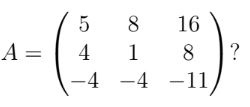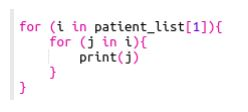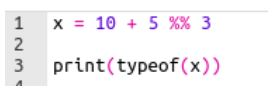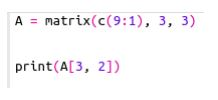# [Week 1 to 8] NPTEL Data Science For Engineers Assignment Answers 2023

NPTEL Data Science For Engineers Assignment Solutions 2023

## NPTEL Data Science For Engineers Week 8 Assignment Answers 2023

1. According to the built model, the within cluster sum of squares for each cluster is ________ (the order of values in each option could be different):-

8.316061 11.952463 16.212213 19.922437
7.453059 12.158682 13.212213 21.158766
8.316061 13.952463 15.212213 19.922437
None of the above

`Answer :- a`

2. According to the built model, the size of each cluster is _ (the order of values in each option could be different):-

• 13 13 7 14
• 11 18 25 24
• 8 13 16 13
• None of the above
`Answer :- c`

3. The Between Cluster Sum-of-Squares (BCSS) value of the built K-means model is ______ (Choose the appropriate range)

• 100 – 200
• 200 – 300
• 300 – 350
• None of the above
`Answer :- a`

4. The Total Sum-of-Squares value of the built k-means model is _ (Choose the appropriate range)

• 100 – 200
• 200 – 300
• 300 – 350
• None of the above
`Answer :- a`

5. Which of the statement is INCORRECT about KNN algorithm?

• KNN works ONLY for binary classification problems
• If k=1, then the algorithm is simply called the nearest neighbour algorithm
• Number of neighbours (K) will influence classification output
• None of the above
`Answer :- a`

6. K means clustering algorithm clusters the data points based on:-

• Dependent and independent variables
• The eigen values
• Distance between the points and a cluster centre
• None of the above
`Answer :- c`

7. The method / metric which is NOT useful to determine the optimal number of clusters in unsupervised clustering algorithms is

• Scatter plot
• Elbow method
• Dendrogram
• None of the above
`Answer :- a`

8. The unsupervised learning algorithm which aims to partition n observations into k clusters in which each observation belongs to the cluster with the nearest centroid is

• Hierarchical clustering
• K-means clustering
• KNN
• None of the above
`Answer :- b`

## NPTEL Data Science For Engineers Week 7 Assignment Answers 2023

1. Which among the following is not a type of cross-validation technique?

LOOCV
k-fold croos validation
Validation set approach

`Answer :- d`

2. Which among the following is a classification problem?

Predicting the average rainfall in a given month.
Predicting whether a patient is diagnosed with a disease or not.
Predicting the price of a house.
Predicting whether it will rain or not tomorrow.

`Answer :- b, b`

3. Find the accuracy of the model.

0.95
0.55
0.45
0.88

`Answer :- a`

4. Find the sensitivity of the model.

0.95
0.55
1
0.88

`Answer :- c`

5. Under the ‘family’ parameter of glm() function, which one of the following distributions correspond to logistic regression for a variable with binary output?

Binomial
Gaussian
Gamma
Poisson

`Answer :- a`

6. What is the dimension of the dataframe?

(150, 5)
(150, 4)
(50, 5)
None of the above

`Answer :- a`

7. What can you comment on the distribution of the independent variables in the dataframe?

The variables Sepal Length and Sepal Width are not normally distributed
All the variables are normally distributed
The variable Petal Length alone is normally distributed
None of the above

`Answer :- b`

8. How many rows in the dataset contain missing values?

10
5
25
0

`Answer :- d`

9. Which of the following code blocks can be used to summarize the data (finding the mean of the columns PetalLength and PetalWidth), similar to the one given below.

lapply(irisdata[, 3:4], mean)
sapply(irisdata[, 3:4], 2, mean)
apply(irisdata[, 3:4], 2, mean)
apply(irisdata[, 3:4], 1, mean)

`Answer :- a, c`

10. What can be interpreted from the plot shown below?

Sepal widths of Versicolor flowers are lesser than 3 cm.
Sepal lengths of Setosa flowers are lesser than 6 cm.
Sepal lengths of Virginica flowers are greater than 6 cm.
Sepals of Setosa flowers are relatively more wider than Versicolor flowers.

`Answer :- b, d`

## NPTEL Data Science For Engineers Week 6 Assignment Answers 2023

1. What is the relationship between the variables, Coupon rate and Bid price?

• Coupon rate = 99.95 + 0.24 * Bid price
• Bid price = 99.95 + 0.24 * Coupon rate
• Bid price = 74.7865 + 3.066 * Coupon rate
• Coupon rate = 74.7865 + 3.066 * Bid price
`Answer :- c`

2. Choose the correct option that best describes the relation between the variables Coupon rate and Bid price in the given data.

• Strong positive correlation
• Weak positive correlation
• Strong negative correlation
• Weak negative correlation
`Answer :- a`

3. What is the R-Squared value of the model obtained in Q1?

• 0.2413
• 0.12
• 0.7516
• 0.5
`Answer :- c`

4. What is the adjusted R-Squared value of the model obtained in Q1?

• 0.22
• 0.7441
• 0.088
• 0.5
`Answer :- b`

5. Based on the model relationship obtained from Q1, what is the residual error obtained while calculating the bid price of a bond with coupon rate of 3?

• 10.5155
• -10.5155
• 6.17
• 0
`Answer :- a`

6. State whether the following statement is True or False.
Covariance is a better metric to analyze the association between two numerical variables than correlation.

• True
• False
`Answer :- b`

7. f R2 is 0.6, SSR=200 and SST=500, then SSE is

• 500
• 200
• 300
• None of the above
`Answer :- c`

8. Linear Regression is an optimization problem where we attempt to minimize

• SSR (residual sum-of-squares)
• SST (total sum-of-squares)
• SSE (sum-squared error)
• Slope
`Answer :- c`

9. The model built from the data given below is Y=0.2x+60 . Find the values for R2 and Adjusted R2 .

• R2 is 0.022 and Adjusted R2 is −0.303
• R2 is 0.022 and Adjusted R2 is −0.0303
• R2 is 0.022 and Adjusted R2 is 0.303
• None of the above
`Answer :- a`

10. Identify the parameters β0 and β1 that fits the linear model β0+β1x using the following information: total sum of squares of X,SSXX=52.53,SSXY=52.01 , mean of X,X¯=4.46 , and mean of Y,Y^=6.32 .

• 1.9 and 0.99
• 10.74 and 1.01
• 4.42 and 1.01
• None of the above
`Answer :- a`

## NPTEL Data Science For Engineers Week 5 Assignment Answers 2023

1. The values of μ1,μ2 and μ3 while evaluating the Karush-Kuhn-Tucker (KKT) condition with all the constraints being inactive are

• μ1=μ2=μ3=1
• μ1=μ2=μ3=0
• μ1=μ3=0,μ2=1
• μ1=μ2=0,μ3=1
`Answer :- b`

2. Gradient based algorithm methods compute

• only step length at each iteration
• both direction and step length at each iteration
• only direction at each iteration
• none of the above
`Answer :- b`

3. The point on the plane x+y−2z=6 that is closest to the origin is

• (0,0,0)
• (1,1,1)
• (−1,1,2)
• (1,1,−2)
`Answer :- d`

4. Find the maximum value of f(x,y)=49−x2−y2 subject to the constraints x + 3y= 10.

• 49
• 46
• 59
• 39
`Answer :- d`

5. The minimum value of f(x,y)=x2+4y2−2x+8y subject to the constraint x+2y=7 occurs at the below point:

• (5,5)
• (−5,5)
• (1,5)
• (5,1)
`Answer :- d`

6. Which of the following statements is/are NOT TRUE with respect to the multi variate optimization?

I – The gradient of a function at a point is parallel to the contours
II- Gradient points in the direction of greatest increase of the function
III – Negative gradients points in the direction of the greatest decrease of the function
IV – Hessian is a non-symmetric matrix

• I
• II and III
• I and IV
• III and IV
`Answer :- c`

7. The solution to an unconstrained optimization problem is always the same as the solution to the constrained one.

True
False

`Answer :- b`

8. A manufacturer incurs a monthly fixed cost of \$7350 and a variable cost,C(m)=0.001m3−2m2+324m dollars. The revenue generated by selling these units is, R(m)=−6m2+1065m. How many units produced every month (m) will generate maximum profit?

(m)=46

(m)=90

(m)=231

(m)=125

`Answer :- b`
`Answer :- a`

## NPTEL Data Science For Engineers Week 4 Assignment Answers 2023

1. Let f(x)=x3+3×2−24x+7. Select the correct options from the following:

x=2 will give the maximum for f(x).
x=2 will give the minimum for f(x).
Maximum value of f(x) is 87.
The stationary points for f(x) are 2 and 4.

`Answer :- b, c`

2. Find the gradient of f(x,y)=x2y at (x,y)=(1,3) .

∇f=
∇f=
∇f=
∇f=

`Answer :- b`

3. Find the Hessian matrix for f(x,y)=x2y at (x,y)=(1,3).

∇2f=
∇2f=
∇2f=
∇2f=

`Answer :- c`

4. Let f(x,y)=−3×2−6xy−6y2. The point (0,0)is a

maxima
minima

`Answer :- b`

5. For which numbers b is the matrix A=[1bb9] positive definite?

−3<b<3
b=3
b=−3
−3b≤3

`Answer :- a`

6. Consider f(x)=x3−12x−5. Which among the following statements are true?

f(x)is increasing in the interval (−2,2).
f(x)is increasing in the interval (2,∞).
f(x)is decreasing in the interval (−∞,−2).
f(x)is decreasing in the interval (−2,2).

`Answer :- b,  d`

7. Consider the following optimization problem:

4×3+21×2+10x−17=0
12×2+42x+10=0
12×2+42x+10>0
12×2+42x+10<0

`Answer :- d`

8. In optimization problem, the function that we want to optimize is called

Decision function
Constraints function
Optimal function
Objective function

`Answer :- d`

9. The optimization problem minx f(x) can also be written as maxx f(x).

True
False

`Answer :- b`

10. Gradient descent algorithm converges to the local minimum.

True
False

`Answer :- a`

## NPTEL Data Science For Engineers Week 3 Assignment Answers 2023

A six sided die is marked ‘1’ on one face, ‘2’ on two of its faces, and ‘3’ on the remaining three faces. The die is thrown twice. Let X denotes the total score in two throws.
Based on the above information, answer questions (1), (2).

1. Find the value of P(X>2.5|X<5). (Enter the answer correct to 2 decimal places).

`Answer :- 0.86`

2. Find the expected value of X.

`Answer :- 3.22`

3. Suppose X ∼ Normal(μ,4). For n=20 iid samples of X, the observed sample mean is 5.2. What conclusion would a z-test reach if the null hypothesis assumes μ=5 (against an alternative hypothesis μ≠5) at a significance level of α=0.05?
Use F−1z(0.025)=−1.9599

• Accept H0
• Reject H0
`Answer :- a`

4. A pharmaceutical company is testing a new drug. The probability that a patient experiencing a side effect from this drug is 0.100.10. If the drug is given to 55 patients, what is the probability that more than 1 patient will experience the side effect? (Enter the answer correct to 22 decimal places.)

`Answer :- 0.08`

5. Suppose X∼Normal(μ,9)(μ,9). For n=100 iid samples of X, the observed sample mean is 11.811.8. What conclusion would a z-test reach if the null hypothesis assumes μ=10.5μ (against an alternative hypothesis μ≠10.5μ≠10.5)?

• Accept H0 at a significance level of 0.10.
• Reject H0 at a significance level of 0.10.
• Accept H0 at a significance level of 0.05.
• Reject H0 at a significance level of 0.05.
`Answer :- b, d`

6. Let X and Y be two independent random variables with Var(X)=9 and Var(Y)=3, find Var(4X−2Y+6).

• 100
• 140
• 156
• None of the above
`Answer :- c`

7. The correlation coefficient of two random variable X and Y is −14, their variance is given by 3and 5. Compute Cov(X,Y).

• -0.854
• 0.561
• -0.968
• None of the above
`Answer :- c`

8. A sample of N observations are independently drawn from a normal distribution. The sample variance follows

• Normal distribution
• Chi-square with N degrees of freedom
• Chi-square with N−1 degrees of freedom
• t-distribution with N−1 degrees of freedom
`Answer :- c`

9. A car manufacturer purchases car batteries from two different suppliers. Supplier X provides 55% of the batteries and supplier Y provides the rest. 5%5% of all batteries from supplier X are defective and 4% of all batteries from supplier Y are defective. You select a battery from the bulk and you found it to be defective. What is the probability that it is from Supplier X?

• 0.0455
• 0.455
• 0.0275
• 0.018
`Answer :- c`

10. Find the t-statistic for the sample data, given that the population mean of the distribution is 8.

• -3.155
• 8.33
• -2.99
• None of the above
`Answer :- a`

## NPTEL Data Science For Engineers Week 2 Assignment Answers 2023

1. Are the vectors [−2 4], [7 −2] and [3 −6] linearly independent?

• Yes
• No
`Answer :- b`

2. Does the set, S={(1,1),(1,2)} spans R2?

• Yes
• No
`Answer :- a`

3. Consider the following system of linear equations of the form Ax=b:1
2x−3y+6z=14
x+y−2z=−3
Which among the following are correct?

• [ 1−4 0 ] is a solution to Ax=b
• [ 0 2 1 ] is a solution to Ax=b
• [ 1 −4 0 ] is a solution to Ax=0
• [ 0 2 1 ] is a solution to Ax=0

[ihc-hide-content ihc_mb_type=”show” ihc_mb_who=”1,2,3″ ihc_mb_template=”1″ ]

`Answer :- a, d`

Consider the following systerm of linear equation:
x+y+z=−2
x+2y−z=1
2x+ay+bz=2

4. Find the conditions on a and b for which the above system has no solution.

• 2a+b−6=0
• a≠4, 2a+b−6= 0
• a=4, b = −2
• 2a+b−6 ≠ 0
`Answer :- b`

5. Find the conditions on a and b for which the above system has a unique solution.

• 2a+b−6=0
• a≠4, 2a+b−6= 0
• a=4, b = −2
• 2a+b−6 ≠ 0
`Answer :- d`

6. Find the conditions on a and b for which the above system has infite number of solutions.

• 2a+b−6=0
• a≠4, 2a+b−6= 0
• a=4, b = −2
• 2a+b−6 ≠ 0
`Answer :- c`

7. Identify the number of free variable from the above rwo echelon matrix.

• 0
• 1
• 2
• 3
`Answer :- b`

8. Which among the following is correct for the above system Ax= b?

• It has infinite number of solutions.
• It has a unique solution.
• It has no solution.
`Answer :- a`

9. For what values of a are matrix

not invertible?

• a=1
• a=−2
• a=−1
• a=2
`Answer :- b, c`

10. Which among the following is true for the determinant of a matrix?

• The determinant of a diagonal matrix is the product of its diagonal entries.
• If one row of a matrix is a scalar multiple of another, the determinant is 1.
• If one row of a matrix is a scalar multiple of another, the determinant is 0.
• The determinant of a permutation matrix can only be 1.
`Answer :- a, c`

11. Which among the following are the eigenvalues of matrix• 1, 3, −3
• 1, 3, 3
• −1, 3, 3
• 1, −3, −3
`Answer :- d`
`Answer :- 2`

13. Let A= [−1 2 2]. Suppose the eighen values corresponding to AAT are a,b and c, then find the value of ab+bc+ca.

• 9
• 0
• 81
• 18
`Answer :- b`

[/ihc-hide-content]

## NPTEL Data Science For Engineers Week 1 Assignment Answers 2023

1. Which of the following variable names are INVALID in R?

• 1_variable
• variable_1
• _variable
• variable@
```Answer :- a. 1_variable
d. variable@

In R, variable names must follow certain rules and conventions. The valid variable names in R can only contain letters (a-z, A-Z), numbers (0-9), and dots (.) or underscores (_). Variable names cannot start with a number or contain special characters other than dots or underscores.```

2. The function ls() in R will

• set a new working directory path
• list all objects in our working environment
• display the path to our working directory
• None of the above
```Answer :- b. list all objects in our working environment

ls() stands for "list objects" and is used to display the names of objects (variables, functions, etc.) that are currently present in the working environment in R. It does not change the working directory or display the path to the working directory. Instead, it lists the objects available in the current R session.```

Consider a following code snippet. Based on this, answer questions 3 and 4.

3. Which of the following command is used to access the value “Shyam”?

• print(patient list)
• print(patient list[])
• print(patient list[])
• print(patient list[])
```Answer :- c. print(patient_list[])

In R, double square brackets [[ ]] are used to extract elements from a list. The first index [] selects the third element of the list, which is itself another list. Then, the second index  selects the second element from this inner list, which is "Shyam". Therefore, patient_list[] will give you the value "Shyam".```

4. The output of the code given below isd. Code will throw an error.

`Answer :- c. `

5. What is the output of following code?• double
• integer
• list
• None of the above
`Answer :- a. double`

6. State whether the given statement is True or False.
The library reshape2 is based around two key functions named melt and cast.

• True
• False
```Answer :- True.

The statement is true. The library reshape2 in R is built around two key functions: melt and dcast (formerly known as acast). These functions are used for reshaping data from wide to long format and vice versa. The melt function is used to transform data from a wide format to a long format, while dcast is used to transform data from a long format to a wide format.```

7. What is the output of following code?• 6
• 4
• 2
• 8
`Answer :- b. 4`

Create the data frame using the code given below and answer questions 8 and 9.

student data = data.frame(student id=c(1:4), student name=c(‘Ram’,‘Harish’,‘Pradeep’,‘Rajesh’))

8. Choose the correct command to add a column named student_dept to the dataframe student_data.

• student datastudent dept=c(“Commerce”, “Biology”, “English”, “Tamil”)
• student data[“student dept”]= c(“Commerce”,“Biology”, “English”,“Tamil”)
• student dept= student data[c(“Commerce”,“Biology”,“English”,“Tamil”)]
• None of the above
```Answer :- a. student_data\$student_dept = c("Commerce", "Biology", "English", "Tamil")

b. student data[“student dept”]= c(“Commerce”,“Biology”, “English”,“Tamil”)```

9. Choose the correct command to access the element Tamil in the dataframe student_data

• student data[]
• student data[]
• student data[]
• None of the above
```Answer :- student_data[]

In R, double square brackets [[ ]] are used to extract elements from a list or dataframe. In this case, student_data[] extracts the third column (which is a vector containing "Commerce", "Biology", "English", "Tamil"), and then  is used to access the fourth element in that vector, which is "Tamil". Therefore, student_data[] will give you the value "Tamil".```

10. The command to check if a value is of numeric data type is _____.

• typeof()
• is.numeric()
• as.numeric()
• None of the above
```Answer :- is.numeric()

The is.numeric() function in R is used to determine whether a given value is of numeric data type or not. It returns TRUE if the value is numeric and FALSE otherwise. For example:```
Scroll to Top